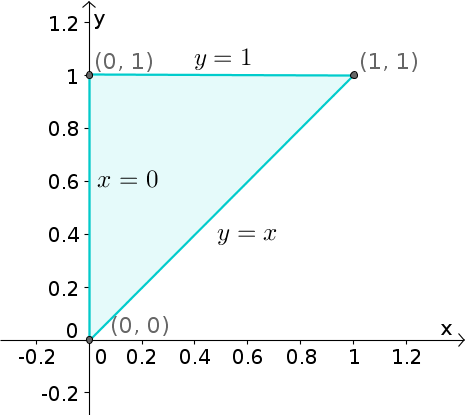# Math Insight

### Image: Triangular region determined by the shadow methodThe triangular region bounded by the lines $x=0$, $y=1$, and $y=x$ is the shadow of a three-dimensional region calculated with the sun being at the positive $z$-axis. The three-dimensional region was the one bounded by the vertical planes $x=0$, $y=1$, and $y=x$ and the angled planes $z=2+x-y$ and $z=x-y$.